# CBSE Class 10 Maths Notes & Important Questions Chapter 11 Constructions

## CBSE Class 10 Maths Notes Chapter 11 Constructions

CBSE Class 10 Maths Notes Chapter 11: Constructions

1. Introduction to Constructions
• Constructions in mathematics refer to drawing geometric figures using a ruler and compass.
• Some of the commonly used constructions include drawing a line parallel to another line, constructing perpendicular bisectors and angle bisectors, and constructing triangles given certain conditions.
1. Basic Constructions
• Bisecting a line segment: Draw an arc with center at one end of the line segment and radius greater than half the length of the segment. Repeat the same with the center at the other end of the segment. The two arcs will intersect at a point. Draw a line passing through this point and the midpoint of the segment. This line will bisect the segment.
• Constructing an angle bisector: Draw an arc with the vertex of the angle as center. The arc should intersect both sides of the angle. Draw another arc with the same radius, this time with the point of intersection of the previous arc and one of the sides of the angle as center. This arc should also intersect the other side of the angle. Draw a line passing through the vertex of the angle and the point of intersection of the two arcs. This line will bisect the angle.
• Constructing a perpendicular bisector: Draw an arc with center at one end of the segment and radius greater than half the length of the segment. Repeat the same with the center at the other end of the segment. The two arcs will intersect at two points. Draw a line passing through these two points. This line will be the perpendicular bisector of the segment.
1. Some Other Constructions
• Constructing a triangle given the lengths of its three sides: Draw a line segment with length equal to the first side. Using the endpoint of this segment as center, draw an arc with radius equal to the length of the second side. Using the other endpoint of the segment as center, draw another arc with radius equal to the length of the third side. The two arcs will intersect at two points. Draw a line passing through one of these points and the endpoint of the original segment. This line will form the required triangle.
• Constructing a triangle given the lengths of one side and two angles: Draw a line segment with length equal to the given side. At one end of the segment, draw an angle equal to one of the given angles. At the other end of the segment, draw an angle equal to the other given angle. Draw a line passing through the two endpoints of the angles. This line will form the required triangle.
1. Tips for Constructions
• Always use a sharp pencil and a good quality compass and ruler.
• Double check your measurements and constructions before drawing the final figure.
• Be patient and take your time while making constructions.

## CBSE Class 10 Maths Important Questions Chapter 11 Constructions

1. Construct a triangle ABC in which AB = 6 cm, ∠B = 45° and BC = 4 cm.
2. Construct an equilateral triangle ABC of side 4.4 cm.
3. Construct a triangle ABC in which AB = 4 cm, BC = 5 cm and ∠ABC = 60°.
4. Construct a parallelogram ABCD in which AB = 6 cm, BC = 5 cm and ∠ABC = 120°.
5. Construct a triangle PQR in which QR = 6 cm, PQ – QR = 2.5 cm and PR – QR = 3.5 cm.
6. Construct a right triangle ABC in which AB = 6 cm, BC = 8 cm and ∠B = 90°.
7. Construct a square ABCD of side 5 cm.
8. Construct a rectangle ABCD in which AB = 6 cm and BC = 5 cm.
9. Construct a rhombus ABCD in which AB = 5 cm and diagonal AC = 8 cm.
10. Construct a trapezium ABCD in which AB || DC, AB = 4 cm, CD = 6 cm and AD = BC = 5 cm.

Note: Please note that the instructions for construction may vary from question to question.

## CBSE Class 10 Maths Important Questions Answers Chapter 11 Constructions

1. Explain the steps to construct an angle of 60 degrees using a compass and ruler.

Solution:

To construct an angle of 60 degrees using a compass and ruler, follow these steps:

Step 1: Draw a line segment AB using a ruler.

Step 2: Place the compass pointer at point A and draw an arc that intersects AB at point P.

Step 3: Without changing the compass width, place the compass pointer at point P and draw another arc that intersects the previous arc at point Q.

Step 4: Draw a line segment PQ using a ruler.

Step 5: Place the compass pointer at point P and adjust the width to point Q. Draw an arc that intersects PQ at point R.

Step 6: Draw a line segment PR using a ruler.

Angle PQR is the required angle of 60 degrees.

1. Construct an isosceles triangle whose base is 8 cm and altitude is 6 cm.

Solution:

To construct an isosceles triangle whose base is 8 cm and altitude is 6 cm, follow these steps:

Step 1: Draw a line segment AB of length 8 cm using a ruler.

Step 2: Draw a perpendicular bisector of AB using a compass and ruler. Mark the intersection of the perpendicular bisector with AB as C.

Step 3: Place the compass pointer at point C and draw an arc of radius 6 cm that intersects the perpendicular bisector at points D and E.

Step 4: Join points A and D, and B and E using a ruler.

Triangle ADB and triangle BEC are the required isosceles triangles.

1. Construct a triangle ABC with AB = 7 cm, BC = 5 cm, and ∠B = 60 degrees.

Solution:

To construct a triangle ABC with AB = 7 cm, BC = 5 cm, and ∠B = 60 degrees, follow these steps:

Step 1: Draw a line segment AB of length 7 cm using a ruler.

Step 2: Draw an angle of 60 degrees at point B using a protractor.

Step 3: Draw a line segment BC of length 5 cm that intersects the angle at point C.

Step 4: Join points A and C using a ruler.

Triangle ABC is the required triangle.

1. Construct a triangle PQR with PQ = 6 cm, QR = 8 cm, and PR = 10 cm.

Solution:

To construct a triangle PQR with PQ = 6 cm, QR = 8 cm, and PR = 10 cm, follow these steps:

Step 1: Draw a line segment PQ of length 6 cm using a ruler.

Step 2: Draw a line segment PR of length 10 cm that intersects PQ at point S.

Step 3: Draw an arc of radius 8 cm with center at point S that intersects PR at points R and T.

Step 4: Join points Q and R, and points P and T using a ruler.

Triangle PQR is the required triangle.

## CBSE Class 10 Maths Notes Chapter 11 Constructions

Determining a Point Dividing a given Line Segment, Internally in the given Ratio M : N

Let AB be the given line segment of length x cm. We are required to determine a point P by dividing it internally in the ratio m : n.

Steps of Construction:

• Draw a line segment AB = x cm.
• Make an acute ∠BAX at the end A of AB.
• Use a compass of any radius and mark off arcs. Take (m + n) points A1, A2, … Am, Am+1, …, Am+n along AX such that AA1 = A1A2 = … = Am+n-1 , Am+n
• Join Am+nB.
• Passing through Am, draw a line AmP || Am+nB to intersect AB at P. The point P so obtained is the A required point which divides AB internally in the ratio m : n.Construction of a Tangent at a Point on a Circle to the Circle when its Centre is Known

Steps of Construction:

• Draw a circle with centre O of the given radius.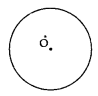• Take a given point P on the circle.
• Join OP.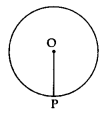• Construct ∠OPT = 90°.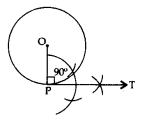• Produce TP to T’ to get TPT’ as the required tangent.Construction of a Tangent at a Point on a Circle to the Circle when its Centre is not Known

If the centre of the circle is not known, then we first find the centre of the circle by drawing two non-parallel chords of the circle. The point of intersection of perpendicular bisectors of these chords gives the centre of the circle. Then we can proceed as above.

Construction of a Tangents from an External Point to a Circle when its Centre is Known

Steps of Construction:

• Draw a circle with centre O.
• Join the centre O to the given external point P.
• Draw a right bisector of OP to intersect OP at Q.
• Taking Q as the centre and OQ = PQ as radius, draw a circle to intersect the given circle at T and T’.
• Join PT and PT’ to get the required tangents as PT and PT’.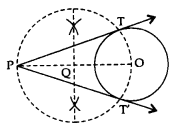Construction of a Tangents from an External Point to a Circle when its Centre is not Known

If the centre of the circle is not known, then we first find the centre of the circle by drawing two non-parallel chords of a circle. The point of intersection of perpendicular bisectors of the chords gives the centre of the circle. Then we can proceed as above.

Construction of a Triangle Similar to a given Triangle as per given Scale Factor mn , m < n.

Let ΔABC be the given triangle. To construct a ΔA’B’C’ such that each of its sides is mn (m < n) of the corresponding sides of ΔABC.

Steps of Construction:

• Construct a triangle ABC by using the given data.
• Make an acute angle ∠BAX, below the base AB.
• Along AX, mark n points A1, A2 …, An, such that AA1 = A1A2 = … = Am-1 Am = … An-1 An.
• Join AnB.
• From Am, draw AmB’ parallel to AnB, meeting AB at B’.
• From B’, draw B’C’ parallel to BC, meeting AC at C’.
Triangle AB’C’ is the required triangle, each of whose sides is mn (m < n) of the corresponding sides of ΔABC.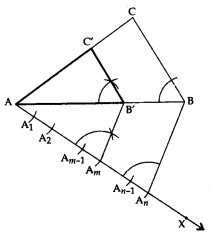Construction of a Triangle Similar to a given Triangle as per given Scale Factor mn , m > n.

Let ΔABC be the given triangle and we want to construct a ΔAB’C’, such that each of its sides is mn (m > n) of the corresponding side of ΔABC.

Steps of Construction:

• Construct a ΔABC by using the given data.
• Make an acute angle ∠BAX, below the base AB. Extend AB to AY and AC to AZ.
• Along AX, mark m points A1, A2 …, An, ..Am, such that AA1 = A1A2 = A2A3 = … = An-1 An = … = Am-1 Am
• Join AnB.
• From Am, draw AmB’ parallel to AnB, meeting AY produced at B’.
• From B’, draw B’C’ parallel to BC, meeting AZ produced at C’.
• Triangle AB’C’ is the required triangle, each of whose sides is (mn) (m > n) of the corresponding sides of ΔABC.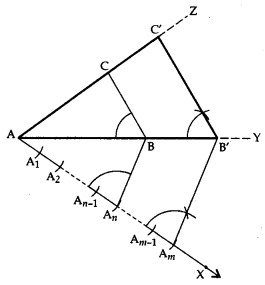Priyanka Mohan (Indian actress) age, bio, career, family, height, unseen images, net-worth, movie, & more Annu Antony (Indian actress) age, bio, career, family, height, unseen images, net-Worth, movie, & more Anu Emmanuel (actress) height, weight, age, boyfriend, biography, unseen images & More Kubbra Sait Birthday: Kubbra Sait used to work as a manager in Microsoft, this is how she entered films Jailer Song:Tamannaah Bhatia sizzles in deep neck top, Vijay Verma also praises her girlfriend
Priyanka Mohan (Indian actress) age, bio, career, family, height, unseen images, net-worth, movie, & more Annu Antony (Indian actress) age, bio, career, family, height, unseen images, net-Worth, movie, & more Anu Emmanuel (actress) height, weight, age, boyfriend, biography, unseen images & More Kubbra Sait Birthday: Kubbra Sait used to work as a manager in Microsoft, this is how she entered films Jailer Song:Tamannaah Bhatia sizzles in deep neck top, Vijay Verma also praises her girlfriend